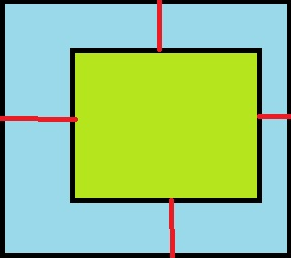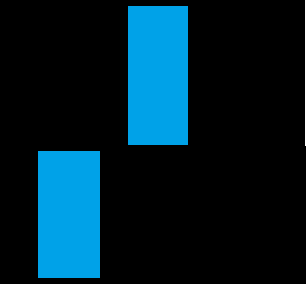# Best Method Of Dynamically Splitting GUI into Geometric Shapes?

Hello Everyone! So not too long ago i was working on a simple script that would makes holes in a Gui(frames) by using a frame as the hole to cut out of another frame. It “works” but i realized a problem, it doesn’t work with more than one frame nor does it work when either the hole( frame representing where a hole should be) or the frame(the Gui the hole is in) Gui is rotated, i found the math to be off in some aspects or at least i didn’t like how i calculated things:

Here is a visualization of what i mean (useful if i didn't explain well):

This is what it does right now (which is great):

Here’s what using multiple frames does( the outcome is no good):

Rotating The Frame:( i believe it’s because i should be making more frames to fill in the gap in between the hole frame and the other parts of the frame( if that makes sense))

Code (warning it's messy)
``````

local FILLING_ = false
local  Runservice = game:GetService("RunService")
local Mouse = game.Players.LocalPlayer:GetMouse()

function MakeLine( Line, StartPoint, EndPoint, ThicknessX,ThicknessY ) ---Make Line
local startX, startY = StartPoint, StartPoint
local endX, endY = EndPoint, EndPoint
Line.AnchorPoint = Vector2.new(0.5, 0.5)
Line.Size = UDim2.new(ThicknessX, ((endX - startX) ^ 2 + (endY - startY) ^ 2) ^ 0.5,0,  ThicknessY)
Line.Position = UDim2.new(0, (startX + endX) / 2, 0, (startY + endY) / 2)
Line.Rotation = math.atan2(endY - startY, endX - startX) * (180 / math.pi)
end

function isTouching(Gui1, Gui2)
local TopLeftGui1 = Gui1.AbsolutePosition
local BottomRightGui1 = TopLeftGui1 + Gui1.AbsoluteSize

local TopLeftGui2 = Gui2.AbsolutePosition
local BottomRightGui2 = TopLeftGui2 + Gui2.AbsoluteSize

return ((TopLeftGui1.x < BottomRightGui2.x and BottomRightGui1.x > TopLeftGui2.x) and (TopLeftGui1.y < BottomRightGui2.y and BottomRightGui1.y > TopLeftGui2.y))
end

function FillAroundObject(lines , Screen,GuiObject )  --Connect Lines, Messy ):

local Start = GuiObject
local GlobalLineSizeY = 0

if isTouching(Start, Screen) then

local AbSolDiffX1 =  (Screen.AbsolutePosition.X  + Screen.AbsoluteSize.X/2) - (Start.AbsolutePosition.X  +  Start.AbsoluteSize.X/2)
local AbSolDiffY1 = ( (Screen.AbsolutePosition.Y  +  Screen.AbsoluteSize.Y/2) - (Start.AbsolutePosition.Y  +  Start.AbsoluteSize.Y/2))

if   (  (Start.AbsolutePosition.Y  +  Start.AbsoluteSize.Y )- (Screen.AbsolutePosition.Y + Screen.AbsoluteSize.Y)  )  < 1 then
MakeLine( lines.B,  {  (Start.AbsolutePosition.X  +  Start.AbsoluteSize.X/2) + AbSolDiffX1 ,   Start.AbsolutePosition.Y  +  Start.AbsoluteSize.Y }  , {  (Start.AbsolutePosition.X + Start.AbsoluteSize.X/2) + AbSolDiffX1 , Screen.AbsolutePosition.Y + Screen.AbsoluteSize.Y  }   , 0 , Screen.AbsoluteSize.X  )
lines.B.Visible = true

else
lines.B.Visible = false
end

if (Start.AbsolutePosition.X - Screen.AbsolutePosition.X ) > 1 then
MakeLine( lines.L1,  {  Start.AbsolutePosition.X ,   (Start.AbsolutePosition.Y  +  Start.AbsoluteSize.Y/2)  + AbSolDiffY1      }  , {   Screen.AbsolutePosition.X  , (Start.AbsolutePosition.Y +  Start.AbsoluteSize.Y/2 ) + AbSolDiffY1  }   , 0 , Screen.AbsoluteSize.Y  )
lines.L1.Visible = true
else
lines.L1.Visible = false
end

if   (Start.AbsolutePosition.X + Start.AbsoluteSize.X  )- (Screen.AbsolutePosition.X  + Screen.AbsoluteSize.X ) < 1 then
MakeLine( lines.L2,  {  Start.AbsolutePosition.X  + Start.AbsoluteSize.X   ,   (Start.AbsolutePosition.Y  +  Start.AbsoluteSize.Y/2)  +   AbSolDiffY1  }  , {   Screen.AbsolutePosition.X + Screen.AbsoluteSize.X  ,  (Start.AbsolutePosition.Y +  Start.AbsoluteSize.Y /2 )  +   AbSolDiffY1 }   , 0 , Screen.AbsoluteSize.Y   )

lines.L2.Visible = true
else

lines.L2.Visible = false
end

if (Start.AbsolutePosition.Y - Screen.AbsolutePosition.Y ) > 1 then
MakeLine( lines.T,  {  ( Start.AbsolutePosition.X  +  Start.AbsoluteSize.X/2) + AbSolDiffX1  ,   Start.AbsolutePosition.Y  }  , { ( Start.AbsolutePosition.X + Start.AbsoluteSize.X/2 ) + AbSolDiffX1    , Screen.AbsolutePosition.Y   }   , 0 , Screen.AbsoluteSize.X)
lines.T.Visible = true

else
lines.T.Visible = false

end

end

end

function Splice(Obj, Screen,Color)
local Handler = Instance.new("Folder", script.Parent)
local L1, L2, T, B = Instance.new("Frame", Handler),  Instance.new("Frame", Handler),  Instance.new("Frame", Handler),  Instance.new("Frame", Handler)
L1.Name = "L1"
L2.Name = "L2"
T.Name = "T"
B.Name = "B"
L1.BorderSizePixel = 0
L2.BorderSizePixel = 0
T.BorderSizePixel = 0
B.BorderSizePixel = 0
L1.BackgroundColor3 = Color
L2.BackgroundColor3 = Color
B.BackgroundColor3 = Color
T.BackgroundColor3 = Color
L1.AnchorPoint = Vector2.new(0.5,0.5)
L2.AnchorPoint = Vector2.new(0.5,0.5)
T.AnchorPoint = Vector2.new(0.5,0.5)
B.AnchorPoint = Vector2.new(0.5,0.5)

FillAroundObject(Handler, Screen, Obj)
end

function GetCollidingGui(Screen, Obj)

for i,v in ipairs(Screen) do
if v and v~= Obj  and v:IsA("Frame") then
if  isTouching(v,Obj ) and v.BackgroundTransparency <1 then
Splice(Obj, v, Color3.fromRGB(44, 223, 12) )
v.BackgroundTransparency = 1

end
end
end

end

GetCollidingGui(script.Parent:GetDescendants(), script.Parent.Obj)

``````

A Brief explanation of the original method i used: Essentially, the way i did things was i created four frames, made them into lines and i connected each side of lets say Part A ( Gui object that would represent the hole ) to the adjacent/corresponding side of the Gui it was inside(Part B). Then i would determine the size according to size of PartB( the gui that i was putting a hole in).So, now that brings me to my question, if there are any other methods or algorithms i can look into to split a 2D based Gui object into Geometric Shapes(squares, triangles, quadrilaterals in general etc.) , what are they and how could i possibly apply them to Roblox ?

Examples of what i somewhat want to achieve

Blue: Is where the holes are
Black: Are the actual frames, as a result of splitting up a GUIHere is a dev forum posts similar to what i am trying to replicate:
Negative GUI elements - #3 by FearMeIAmLag

Note: i am more or less seeking the math and logic behind calculating what shapes, size and positioning are needed to fill in a 2d shape(in this case mostly quadrilaterals)! Also by Dynamically i mean allowing the Gui and Hole Size to be manipulated, but still have the areas around the hole be freely adjusted(automatically).

Any Help, comments or thoughts are appreciated, even if you tell me that it’s practically impossible to do in Roblox then feel free to do so, if you have any questions please let me know!

(Ps: i know that Roblox is limited as far as gui shapes go, that’s why i think methods using quadrilaterals will only really work for splitting GUI objects up)

Thank you for reading, sry for such a long post…

1 Like

The simplest method would be to use simplexes. Simplexes are easy to create to fill any shape. The 2D simplex is the triangle. Now we can’t make a whole bunch of triangle images for every possible angle, but luckily we don’t have to. Every triangle can be broken into two right triangles by splitting the hypotenuse with a perpendicular line to the opposite corner. This method is used in all triangle terrain implementations.

Splitting the original frames into triangles is trivial by splitting on the diagonal. To make a hole in a shape, find the intersection of the shapes. Points from the hole inside the frame are added, and points from the frame inside the hole are removed. Lastly, lines on the exterior of the hole and exterior of the frame that intersect form two new points which will be connected to the points added by the points of the hole that were added on the interior of the frame. The added points maintain their connections to each other. Once the boundaries are known, you can use a sweeping line approach to reform triangles. Optimally, you would only need to reform the parts of the frame whose triangles had one or more points change. Sweep a line from one direction to another (say the x axis) and keep track of the points. Every third point form a triangle and remove the oldest point. Since this requires a priority queue it is O(n * log(n)) time and O(n) space. You can find my binary minimum heap implementation here:

6 Likes

After a bit of researching i decided to use the Deuluany Algorithim for the time being instead of a sweeping line, and after playing around for a while i got a functional (with issues, there is a problem with making 2d triangles to fill the area ) result: https://devforum.roblox.com/t/what-are-you-working-on-currently-2020/419774/73?u=jaycbee05

Thank you for pointing me in the right direction!

I will probably end up using a sweeping line eventually, I have heard its pretty fast than some other methods

1 Like